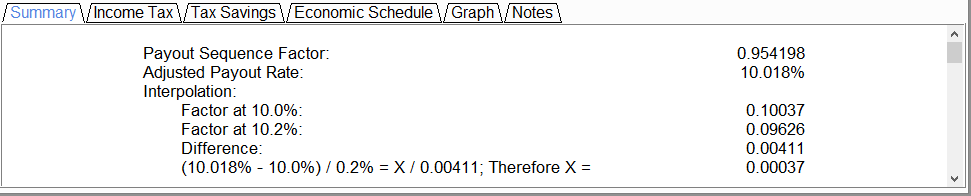Forums Support Library CFP – Charitable Financial Planner Charitable Remainder Unitrust Summary Report

Tagged: ,

• Author
Posts
•The fair market value of a remainder interest in a charitable reminder unitrust is its present value. The present value is determined under Treas. Reg. §1.664-4(a)(1).

An adjusted payout rate is determined by multiplying the fixed percentage payout by the appropriate payout sequence factor. The payout sequence factor can be found in the IRS Publication 1458, Actuarial Values Beta Volume. If the adjusted payout rate is between the rates shown in the table, a linear interpolation is required.

When the remainder factor is computed, the present value of the remainder interest is obtained by multiplying the factor times the fair market value of the trust assets.

The summary tab shows the direct results from the values that are entered into the input fields.

If a calculation is of the “Life” or “Shorter” type, if there is more than one noncharitable beneficiary, and if their interests are all “vested” and not subject to revocation by the grantor, then there may be a taxable gift equal to the present value of all noncharitable interests other than any interests retained by the grantor. The program assumes that the first measuring life is that of the grantor and shows the value of all succeeding noncharitable interests, which is calculated by subtracting the present value of the interests retained by the grantor (calculated using only the first measuring life) from the total present value of all noncharitable interests.

• Payout Sequence Factor: It is used to calculate the payout rate (the frequency of payments). The factor is found in Table F(1) (for transfers prior to May 1989) or Table F for the appropriate interest rate (for transfers after April 1989) that corresponds to the number of months by which the valuation date precedes the payout date. The tables can be found in the IRS Publication 1458, Actuarial Values Beta Volume.
• Adjusted Payout Rate: An adjusted payout rate is determined by multiplying the fixed percentage payout by the appropriate payout sequence factor. If the adjusted payout rate is between the rates shown in the table, a linear interpolation is required.
• Term of Years Remainder Interest: The present value of the remainder interest is obtained by multiplying the factor times the fair market value of the trust assets. A number that is derived from IRS tables depending on the term of years.
• Present Value of Remainder Interest: The present value of remainder interest is its fair market value. The present value is determined under Treasury Regulations §1.664-4(a)(1).
• Donor’s Deduction: The dollar amount that is not subject to taxation (income tax).
• Donor’s Deduction as Percentage of Amount Transferred: The percentage amount that is not subject to taxation (income tax).